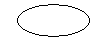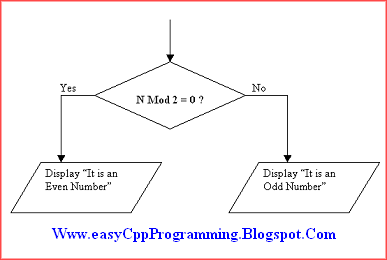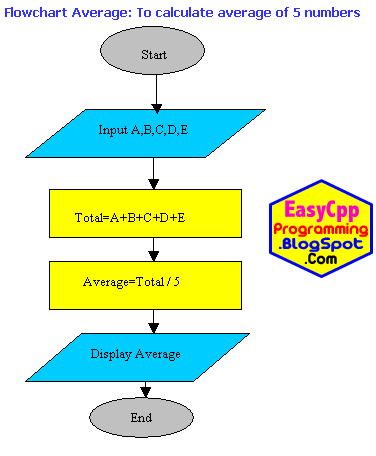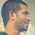# C++ Tutorial With Basic Programs for Beginners

C++ tutorial, C++ programs, C++ code, C++ flowcharts, C++ statements, C++ syntax, C++ examples

## Tuesday, July 12, 2011

### Definition of Flowchart

Today we will discuss The Role of Flowcharts in Computer Programming. Flowchart is the graphical representation of an algorithm. It is used to show all the steps of an algorithm in a sequence pictorially. An algorithm may be converted to a flowchart. The flowchart is then converted into a program written in any programming language.Importance and Symbols of Flowchart in Computer Programming

### Advantages of Flowcharts

So what are the main advantages of using Flowcharts in Computer Programming?
Flowchart is used for the following reasons:
1. Flowchart represents an algorithm in graphical symbols.
2. It shows the steps of an algorithm in an easy way.

3.
3. It is used to understand the flow of the program graphically.
4. Flowchart can be easier and more effective to understand the logic of the given program instantly.
5. A flowchart will be helpful to the programmer during coding / program development phase.

### Commonly Used Symbols To Draw a Flowchart

So after reading the benefits of using Flowcharts in Computer programming, we will learn how to draw a flow chart. Following are the common symbols used in Flowchart:

#### 1. Start/End

Oval symbol is used to represent the start or end of the flowchart. Every program has a Start and End. So we will add an Oval symbol with caption "Start" in the beginning of each flow chart. Similarly, we will place an Oval symbol with the tag "End" or "Stop" at the end of every flow chart.#### 2. Input/Output

Parallelogram symbol is used to represent an input or output step. So if we wish to input the value of a variable N in our program, we will put a parallelogram symbol there with a caption of "Input N" or "Read N" etc. Similarly, we will use this symbol to represent output statements in program too. For Example, if we encounter an output statement in our program algorithm to show a message "Enter a Number:", we will add a parallelogram symbol with the caption "Enter a Number". If we wish to show the value of a variable, we may add parallelogram symbol with the text "The result =", result. Where "result" is a variable name.

#### 3. Process

Rectangle symbol is used to represent a process step. A process may be a calculation or assignment etc. The algorithmic statements like Calculate Sum = Num1 + Num2 can be represented by the rectangle symbol in flowcharts. Similarly, the assignment statements like Width = 10 may also be represented by rectangle symbol.

#### 4. Selection

Diamond symbol is used to represent a selection step. A condition is given in the diamond. If condition is true then flow of control will go in one direction. If condition is false then control will go in other direction.
For example, we wish to represent an IF statement in a flowchart, say IF Number N is divisible by 2 Then Display "It is an Even Number" Else Display "It is an Odd Number" End IF In this case the diamond symbol will be used along with parallelogram symbols as shown below:#### 5. Flow Lines

Arrow symbols are used to represent the direction of flow in the flowchart. There are four types of flow lines.

#### 6. Connector

Circle symbol is used to combine different flow lines. It is used as a connector symbol in flow charts.

### Solved Flow Chart Examples:

Flowchart Example 1 Develop a flowchart to input two numbers from the user, calculate its sum and then display the result.Example Add Two Numbers Flowcharts in Computer Programming
Flowchart Example 2 Develop a flowchart to input 5 numbers from the user, calculate its average and then display the result.Example of Average program Flowcharts in Computer Programming

Dear Readers, you just read the role of Flowcharts in Computer Programming in detail with Flow chart definition, symbols, advantages of flow charts and examples of flow charts.

Further Suggested Readings: After Download Free Turbo C++ Ver 3.0 IDE!

Basic Structure of a C++ Program

C++ Program Development Cycle

How To Write, Compile and Run Your First C++ Program

How To Start Turbo C++ IDE

How To Install Turbo C++ 3.0 - IDE

Features of C++ Programming Language

Brief History of C++

Flowcharts in Computer Programming

The Role of Algorithms in Programming

How To Start Computer Programming

What is a Computer Program

- See more at: http://easycppprogramming.blogspot.com
Share:

#### 1 comment:

1.great flowchart tutorial for beginners. It is even better if you have provide with a flowchart software to try drawing flowcharts. I suggest creately diagramming and collaboration software to draw flowcharts.

We Love To Hear From You!